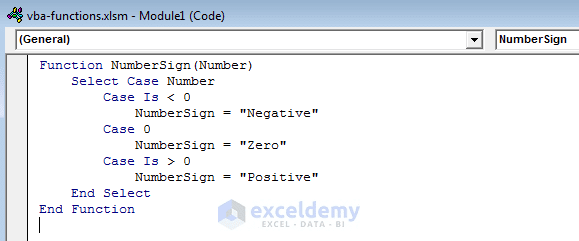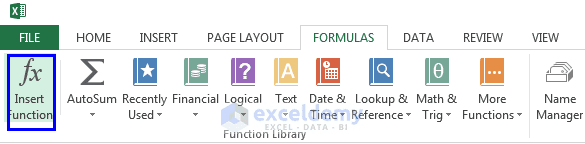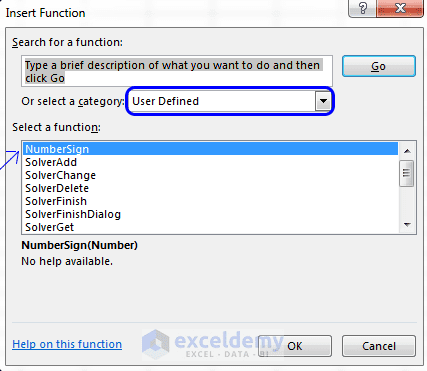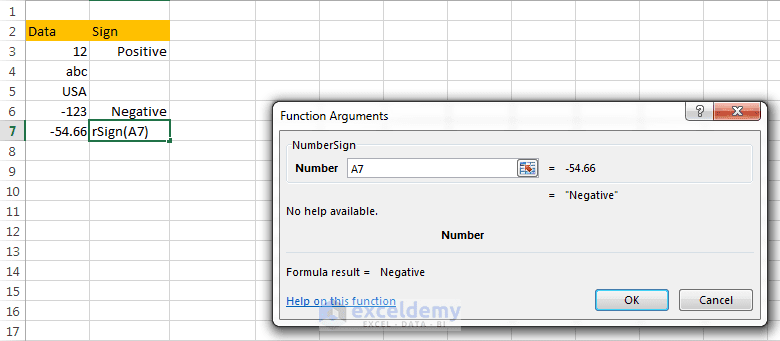Disclosure: This post may contain affiliate links, meaning when you click the links and make a purchase, we receive a commission.

# How to Create Custom VBA functions and Use them in the Worksheet

We already know that there are two types of VBA macros from this article: Two types of VBA Macros: VBA Sub procedures & VBA functions. In our this article, we shall focus closely on how to create custom VBA functions and use them in the worksheet like built-in Excel functions. Some built-in Excel functions are: FIND(), SUMPRODUCT(), OFFSET(), and etc.

## A Synopsis of VBA Functions

Function procedures that we write in VBA can be used in two situations:

• We can call our VBA functions from a different VBA procedure.
• We can also use our VBA functions in a worksheet like we use built-in Excel functions in Excel formulas.

Excel has around 400 built-in and predefined worksheet functions. You may be amazed to think why anyone needs to develop additional functions when Excel has so many ready-to-use functions.

The first and foremost reason is: creating a custom function can simplify and shorten our formulas combining two or more Excel built-in functions. Simple and shorter formulas are easily understandable.

Another important reason is: you can write functions to perform operations that are impossible to perform in other ways.

## Ain’t you familiar with writing code in VB Editor?

If you’re not familiar with entering and editing VBA code in the Visual Basic () Editor, then read these articles: Writing VBA code in Excel: Entering and Editing code and How to Write a Function Procedure in Visual Basic Editor.

## An Introductory Example of VBA function

If you understand VBA, writing code for creating a custom function is easy. Without further ado, let’s explain the things with an example. This function is stored in a VBA module. You can access the VBA module from the VB Editor. Press ALT+F11 to open the VB Editor.

### A custom function

You’re going to create NumberSign function. This function uses one argument.

The function returns a text string. The text string may be:

• Positive: if its argument is greater than zero
• Negative: if its argument is less than Zero
• Zero: if its argument is equal to zero.

The argument may be Non-numeric. In that case, the function will return an empty string. The following figure shows the NumberSign function code.A simple and custom function, NumberSign

We could accomplish the same result using Excel’s IF() predefined function like this:

`=IF(A10=0, "Zero", IF(A10&gt;0, "Positive" , "Negative"))`

You see in this case, custom function is easier to use when compared with predefined Excel function.

### Using NumberSign function in a worksheet

NumberSign function just works like any Excel built-in function. To insert this function in a formula choose Formulas ➪ Function Library ➪ Insert Function Wizard.

Choosing this command will display the Insert Function dialog box. Custom functions are listed in the User Defined category. When you select the function from the list, you can then use the Function Arguments dialog box to specify the arguments for the function, as shown in the figure below. You can use the custom functions with other elements in your Excel formula.Choosing Insert Function Wizard.Insert Function dialog box. Find out your custom functions under User Defined list.

Function Arguments dialog box where you enter the necessary arguments.Entering A4 as an argument to check the sign of the value of A4.

### Analyzing NumberSign(Number) custom function

We’ll analyze the NumberSign function in this section. Here is the code of NumberSign function:

```Function NumberSign(Number)
Select Case Number
Case Is &lt; 0
NumberSign = "Negative"
Case 0
NumberSign = "Zero"
Case Is &gt; 0
NumberSign = "Positive"
End Select
End Function
```

Always the function procedure starts with the keyword Function, followed by the name of the function (NumberSign). NumberSign function uses one argument (Number), and the argument’s name (Number) is enclosed in parentheses.

When the function is used in a worksheet, the argument Number can be a cell reference (such as =NumberSign(A1)) or a literal value (such as =NumberSign(–123)). The function can also be used in another procedure and the argument can be a numeric variable, a literal number, or a value that is obtained from a cell.

The NumberSign function uses the Select Case construct. If Number is less than zero, NumberSign is assigned the text Negative. If Number is equal to zero, NumberSign is Zero. If Number is greater than zero, NumberSign is Positive. The value returned by a function is always assigned to the function’s name.

### Oops! NumberSign function has a bug!

Try this formula in any cell of a worksheet.

`=NumberSign("ABC")`

You will find that this function is resulting value Positive. This is not what you intended. Your target was to check numeric numbers. When the function will deal with non-numeric values, it must show something different. The function has a bug. Let’s add some more line of code to correct this error.

Here is a revised version of NumberSign function that returns an empty string if the argument is non-numeric. We’ve used the VBA IsNumeric() function to check the argument, Number. If the argument, Number, is numeric, the code checks its sign. If the argument is non-numeric, the Else part of the If-Then-Else structure is executed.

```Function NumberSign(Number)
If IsNumeric(Number) Then
Select Case Number
Case Is &lt; 0
NumberSign = "Negative"
Case 0
NumberSign = "Zero"
Case Is &gt; 0
NumberSign = "Positive"
End Select
Else
NumberSign = ""
End If
End Function
```

Without using a custom function, you could easily get the same result using the following formula:

`=IF(ISNUMBER(A1),IF(A1<0, "Negative", IF(A1>0, "Positive", "Zero"))," ")`

vba-functions.xlsm

Happy Excelling ☕